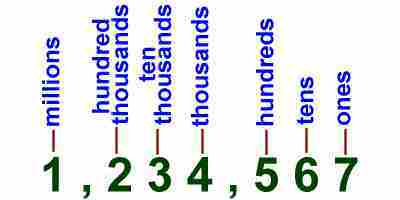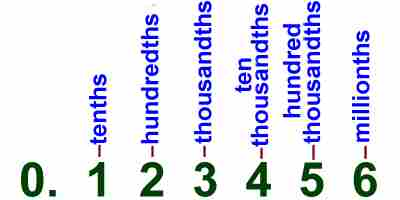# Kids Math

## Decimals Place Value

Summary

We use decimals as our basic number system. The decimal system is based on the number 10. It is sometimes called a base-10 number system. There are other systems that use different base numbers, like binary numbers which use base-2.

Place Value

One of the first things to learn about decimals is the place value. The place value is the position of a digit in a number. It determines the value that the number holds.

Let's take a basic example:

Comparing the numbers 700, 70, and 7; the digit "7" has a different value depending on its place within the number.

7 - ones place
70 - tens place
700 - hundreds place

The place value of the 7 determines the value it holds for the number. As the place moves to the left, the value of the number becomes greater by 10 times.Decimal Point

Another important idea for decimals and place value is the decimal point. The decimal point is a dot between digits in a number. Numbers to the left of the decimal point are greater than 1. Numbers to the right of the decimal point hold values smaller than 1. The right of the decimal point is like a fraction.

Example:

0.7 - tenths
0.07 - hundredths

In the case where the place value is to the right of the decimal point, the place tells you the fraction. For example, 0.7 is in the tenths place and represents the fraction 7/10. In the number 0.07 the 7 is in the hundredths place and is the same as the fraction 7/100.Ten to the Power

In the decimal system each place represents a power of 10. Here is a chart showing how this works.

 Millions 7,000,000 7x106 Hundred thousands 700,000 7x105 Ten thousands 70,000 7x104 Thousands 7,000 7x103 Hundreds 700 7x102 Tens 70 7x101 Ones 7 7x100 Tenths 0.7 7x10-1 Hundredths 0.07 7x10-2 Thousandths 0.007 7x10-3 Ten thousandths 0.0007 7x10-4 Hundred thousandths 0.00007 7x10-5 Millionths 0.000007 7x10-6

For example, when we say 7 is in the hundreds place in the number 700, this is the same as 7x102. You can see from the chart that when the place value is to the right of the decimal point, then the power of 10 becomes negative.

Lining Up Decimals

When you start to do arithmetic with decimals, it will be important to line up the numbers properly. When lining up decimal numbers, be sure to line them up using the decimal point. This way you will have the other place values lined up as well.

Example:

Line up the numbers 2,430 and 12.07.

At first you may want to just write these numbers down like this:

2,430
12.07

However, the decimal points and place values are not lined up. You can rewrite 2,430 with decimal points so that it looks like 2,430.00. Now when you line up the decimal points you get:

 2,430.00 12.07

The two numbers are lined up by place value and you can begin math like adding or subtracting.

Back to Kids Math

Back to Kids Study